# Texas Go Math Grade 5 Lesson 10.2 Answer Key Number Patterns in Tables

Refer to our Texas Go Math Grade 5 Answer Key Pdf to score good marks in the exams. Test yourself by practicing the problems from Texas Go Math Grade 5 Lesson 10.2 Answer Key Number Patterns in Tables.

## Texas Go Math Grade 5 Lesson 10.2 Answer Key Number Patterns in Tables

Unlock the Problem

When the output in a pattern depends on the input, you can write a rule to describe the relationship between inputs and outputs.

Marcus wants to buy light-up balls for a party. They cost $1 each. The store charges the same shipping fee regardless of how many light-up balls are ordered. So, Marcus has to pay for the light-up balls and pay a shipping fee. The table below shows the cost c for w light-tip balls. How much will Marcus pay for 12 light-up balls? Write a rule to describe the pattern in the table.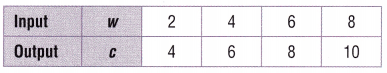STEP 1: Describe the relationship between the number of light-up balls and the cost.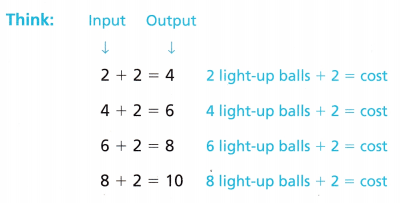The output is _________ more than the input. STEP 2: Decide what operation to use to write a rule. c = _________ + __________ Think: Add 2 to the input to get the output Since the pattern is additive, use addition to write a rule. STEP 3: Use the rule to find the cost of 12 light-up balls, c = w + 2 c = _________ + 2 Replace w with the number of light-up balls. c = _________ Add to find the cost. So, the 12 light-up balls cost$ ________.
Step 1 : The output is 2 more than the input,
Step 2 : The operation used to write a rule is c = w + 2,
Step 3: The 12 light-up balls cost $14, Explanation: Given Marcus wants to buy light-up balls for a party. They cost$1 each.
The store charges the same shipping fee regardless of how many light-up balls are ordered.
So, Marcus has to pay for the light-up balls and pay a shipping fee.
The table above showed the cost c for w light-tip balls.
So Marcus pay for 12 light-up balls is
Step 1: Described the relationship between the number of light-up balls and the cost.The output is 2 more than the input.
Step 2: Decided what operation to use to write a rule as for c,
Thinking: Add 2 to the input to get the output
Since the pattern is additive, use addition to write a rule as
c = w + 2,
Step 3: Used the rule to find the cost of 12 light-up balls,
c = w + 2, Replaced w with the number of light-up balls as 12.
c = 12 + 2 = 14.
So, the 12 light-up balls cost $14. Example Find the rule. Patterns can also involve multiplicationThe output is _________ times the input. The pattern is multiplicative. The rule is y = _________ × _________. Answer: The output is 7 times the input. The pattern is multiplicative, the rule is y = n X 7, Explanation: Step 1:the output is 7 times more than the input. Step 2: Decided what operation to use to write a rule as for y, Thinking: Multiplying 7 to the input to get the output, Since the pattern is multiplicative, using multiplication to write a rule as y = 7n. Share and Show Question 1. Use a rule to describe the pattern in the table.Multiply by _________. Rule: _________ Answer: The output is 9 times the input. The pattern is multiplicative, the rule is t = s X 9, Explanation: Step 1: If input is 1, output is 1 X 9 = 9, if input is 2, output is 2 X 9 = 18, if input is 3, output is 3 X 9 = 27, if input is 4, output is 4 X 9 = 36, the output is 9 times more than the input. Step 2: Decided what operation to use to write a rule as for t, Thinking: Multiplying 9 to the input to get the output, Since the pattern is multiplicative, using multiplication to write a rule as t = s X 9. Decide if the pattern shown in the table is additive or multiplicative. Write a rule to describe the pattern. Question 2.The pattern is _______________ Rule: ______________ Answer: The pattern is additive, Rule is c = a + 3, Explanation: Step 1: If input is 2, output is 2 + 3 = 5, if input is 4, output is 4 + 3 = 7, if input is 6, output is 6 + 3 = 9, if input is 8, output is 8 + 3 = 11, the output is 3 more than the input. Step 2: Decided what operation to use to write a rule as for c, Thinking: Adding 3 to the input to get the output, Since the pattern is additive, using addition to write a rule as c = a + 3. Question 3.The pattern is _______________ Rule: ______________ Answer: The output is 13 times the input. The pattern is multiplicative, the rule is p = r X 13, Explanation: Step 1: If input is 1, output is 1 X 13 = 13, if input is 2, output is 2 X 13 = 26, if input is 3, output is 3 X 13 = 39, if input is 4, output is 4 X 13 = 52, the output is 13 times more than the input. Step 2: Decided what operation to use to write a rule as for p, Thinking: Multiplying 13 to the input to get the output, Since the pattern is multiplicative, using multiplication to write a rule as p = r X 13. Problem Solving Question 4. Write Math Explain how you can find a rule for a pattern in a table. Answer: Checking rule either using the pattern is added, subtracted, multiplied, or divided, Explanation: To determine the rule for a table, simply look at one pair of input/output numbers and figure out what number has been added, subtracted, multiplied, or divided by it. Then, make sure that pattern matches the rest of the numbers. Once we know the rule for a table, we can finish filling in the rest of it. Question 5. H.O.T. Explain whether the formula for the area of a rectangle can be used to show an additive pattern or a multiplicative pattern. Answer: The area of a rectangle can be used to show an multiplicative pattern, Explanation: As the formula for the area of a rectangle is area = length X breadth,(a = l X b) it clearly shows multiplication of length and breadth, therefore the area of a rectangle can be used to show an multiplicative pattern. Problem Solving Question 6. Multi-Step To make soup, Juan adds 3 cups of hot water to each package of dried soup. Write a rule for the pattern. Make a table to determine how many cups of hot water are needed for 18 packages of dried soup. Answer: The output is 3 times the input. The pattern is multiplicative, the rule is c = p X 3,Number of cups c of hot water needed for 18 packages of dried soup is 18 X 3 = 54 cups, Explanation: Given to make soup, Juan adds 3 cups of hot water to each package of dried soup. Wrote a rule for the pattern. as c = p X 3, here c is cups of hot water and p is number of packages, Made a table above to determine how many cups of hot water are needed for 18 packages of dried soup above as Step 1: If input is 1, output is 1 X 3 = 3, if input is 2, output is 2 X 3 = 6, if input is 3, output is 3 X 3 = 9, if input is 4, output is 4 X 3 = 12, If input is 5, output is 5 X 3 = 15, if input is 6, output is 6 X 3 = 18, if input is 7, output is 7 X 3 = 21, if input is 8, output is 8 X 3 = 24, If input is 9, output is 9 X 3 = 27, if input is 10, output is 10 X 3 = 30, if input is 11, output is 11 X 3 = 33, if input is 12, output is 12 X 3 = 36, If input is 13, output is 13 X 3 = 39, if input is 14, output is 14 X 3 = 42, if input is 15, output is 15 X 3 = 45, if input is 16, output is 16 X 3 = 48, If input is 17, output is 17 X 3 = 51, if input is 18, output is 18 X 3 = 54, the output is 3 times more than the input. Step 2: Decided what operation to use to write a rule as for packages p, Thinking: Multiplying 3 to the input to get the output, Since the pattern is multiplicative, using multiplication to write a rule as c = p X 3, therefore number of cups c of hot water needed for 18 packages of dried soup is 18 X 3 = 54 cups. Question 7. H.O.T. Multi-Step Liam and Nora are playing a spelling game. The number of points for each word a player spells correctly is the sum of the number of letters in the word and 3 bonus points for spelling correctly. The table below shows the scoring system. Find the rule and the number of points for spelling an eight-letter word correctly.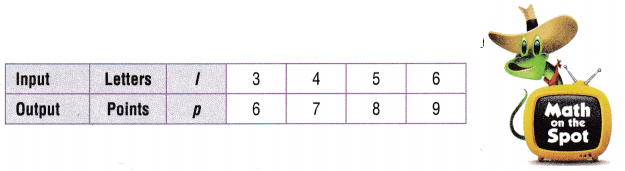Answer: Rule : p = l + 3, Number of points for spelling an eight-letter word correctly is 11, Explanation: Given Liam and Nora are playing a spelling game. The number of points for each word a player spells correctly is the sum of the number of letters in the word and 3 bonus points for spelling correctly. The table showed the scoring system as If input is 3, output is 3 + 3 = 6, if input is 4, output is 4 + 3 = 7, if input is 5, output is 5 + 3 = 8, if input is 6, output is 6 + 3 = 9, therefore the rule is additive pattern as p = l + 3, so the number of points for spelling an eight-letter word correctly is p = 8 + 3 = 11 points. Question 8. H.O.T Apply Kirk is building a storage cabinet. To be sure of having enough wood, he allows an extra 2 inches on measurements. Write a rule for the pattern. Make a table to find the number of inches that Kirk would measure if he wanted an 11-inch board. Answer: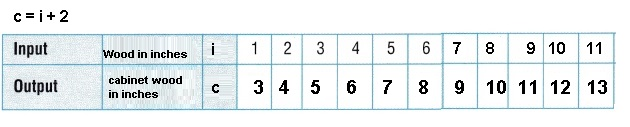Kirk need 11 + 2 = 13 inch measure if he wanted an 11-inch board, Explanation: Given Kirk is building a storage cabinet. To be sure of having enough wood, he allows an extra 2 inches on measurements. if If input is 1, output is 1 + 2 = 3, if input is 2, output is 2 + 2 = 4, if input is 3, output is 3 + 2 = 5 and so on till if input is 11, output is 11 + 2 = 13, The rule for the pattern is c = i + 2, where c is cabinet wood and i is number inches of wood to make cabinet, Made the table above and the number of inches that Kirk would measure if he wanted an 11-inch board is 11 + 2 = 13 inch. Daily Assessment Task Fill in the bubble completely to show your answer. Use the table for 9-10.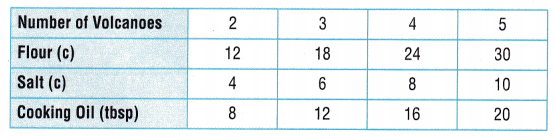Question 9. Analyze Using the recipe shown in the table, how many cups of flour will you need to make 13 model volcanoes? (A) 30 cups (B) 48 cups (C) 78 cups (D) 54 cups Answer: (C) 78 cups, Explanation: Using the recipe shown in the table, number of cups of flour I will need to make 13 model volcanoes is as Number of volcanoes if 2 means flour(c) is 12, if 3 means 18 and so on its rule is c = n X 6 where c is cups of flour and n is number of volcanoes, therefore for 13 we require 13 X 6 = 78 cups of flour which matches with (C). Question 10. If v is the number of volcanoes and s is the number of cups of salt, which of the following rules describes the pattern in the table? (A) v = s + 2 (B) j = n × 2 (C) s = v + 2 (D) s = v × 2 Answer: (D) s = v x 2, Explanation: Using the recipe shown in the table, If v is the number of volcanoes and s is the number of cups of salt, the following rules describes the pattern in the table as if v = 2 then s = v X 2 = 4, if v =3 then s = 3 X 2 = 6 and so on therefore the pattern in the table is s = v X 2 which matches with (D). Question 11. Multi-Step The table shows the shipping boxes received by Snazzy Stuff clothing store. Suppose that the store pays$8 for a shirt.
How much money does the store pay for 10 boxes of shirts?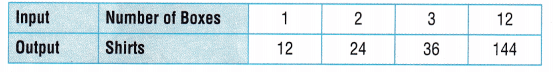(A) $120 (B)$96
(C) $960 (D)$192
(C) $960, Explanation: Given the table shows the shipping boxes received by Snazzy Stuff clothing store. Suppose that the store pays$8 for a shirt.
seeing the table the rule is s = n X 12 where n is number of boxes and
s is number of shirts so for 10 boxes means shirts are 10 X 12 = 120,
now given that the store pays $8 for a shirt so money does the store pay for 10 boxes of shirts is 120 X$8 = $960 which matches with (C). TEXAS Test Prep Question 12. Which of the following rules describes the pattern in the table?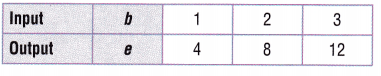(A) e = b + 4 (B) e = b × 4 (C) b = e + 4 (D) b = e × 4 Answer: (B), e = b X 4, Explanation: Given table where input b = 1 output e = 1 X 4 =4, if input b =2 output e = 2 X 4 =8, if input b =3 output e = 3 X 4 =12 therefore the pattern in the table is e = b X 4 which matches with (B). ### Texas Go Math Grade 5 Lesson 10.2 Homework and Practice Answer Key Find the rule to describe the pattern in the table. Decide if the rule is additive or multiplicative. Question 1.The rule is ________________. Rule: ______________ Answer: The rule is n = m X 11, Explanation: Step 1: If input is 1, output is 1 X 11 = 11, if input is 2, output is 2 X 11 = 22, if input is 3, output is 3 X 11 = 33, if input is 4, output is 4 X 11 = 44, the output is 11 times more than the input. Step 2: Decided what operation to use to write a rule as for n, Thinking: Multiplying 11 to the input to get the output, Since the pattern is multiplicative, using multiplication to write a rule as n = m X 11. Question 2.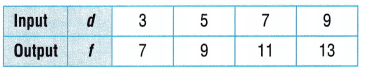The rule is ______________ Rule: _____________ Answer: Rule f = d + 4, Explanation: Step 1: If input is 3, output is 3 + 4 = 7, if input is 5, output is 5 + 4 = 9, if input is 7, output is 7 + 4 = 11, if input is 9, output is 9 + 4 = 13, the output is 4 more than the input. Step 2: Decided what operation to use to write a rule as for f, Thinking: Adding 4 to the input to get the output, Since the pattern is additive, using addition to write a rule as f = d + 4. Question 3.The rule is ______________ Rule: _____________ Answer: The rule is q = p X 15, Explanation: Step 1: If input is 2, output is 2 X 15 = 30, if input is 3, output is 3 X 15 = 45, if input is 4, output is 4 X 15 = 60, if input is 5, output is 5 X 15 = 75, the output is 15 times more than the input. Step 2: Decided what operation to use to write a rule as for q, Thinking: Multiplying 15 to the input to get the output, Since the pattern is multiplicative, using multiplication to write a rule as q = p X 15. Question 4.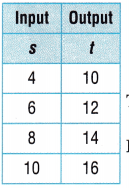The rule is ______________ Rule: _____________ Answer: Rule t = s + 6, Explanation: Step 1: If input is 4, output is 4 + 6 = 10, if input is 6, output is 6 + 6 = 12, if input is 8, output is 8 + 6 = 14, if input is 10, output is 10 + 6 = 16, the output is 6 more than the input. Step 2: Decided what operation to use to write a rule as for f, Thinking: Adding 6 to the input to get the output, Since the pattern is additive, using addition to write a rule as t = s + 6. Problem Solving Question 5. Marcus pours 12 ounces of cranberry juice into a punch bowl. He uses a container to add sparkling water to the bowl one ounce at a time. Write a rule to show the pattern and use it to calculate how many ounces of liquid Marcus will have in the bowl after adding 8 containers of sparkling water. Answer: Number of ounces of liquid Marcus will have in the bowl after adding 8 containers of sparkling water is 8 ounces, Explanation: Given Marcus pours 12 ounces of cranberry juice into a punch bowl. He uses a container to add sparkling water to the bowl one ounce at a time. Rule to show the pattern and use it to calculate how many ounces of liquid Marcus will have in the bowl after adding 8 containers of sparkling water is taking ounces as o and c as containers of sparkling water therefore rule is o = c X one ounce so number of ounces of liquid Marcus will have in the bowl after adding 8 containers of sparkling water is 8 ounces. Lesson Check Fill in the bubble completely to show your answer. Question 6. The winning entry in the inventors’ competition was a robot made with wheels, gears, and bolts. Using the table shown below, how many bolts are needed to make 7 robots?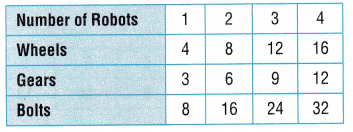(A) 40 (B) 21 (C) 28 (D) 56 Answer: (D) 56, Explanation: The winning entry in the inventors’ competition was a robot made with wheels, gears, and bolts. Using the table shown above if 1 robot with 8 bolts, 2 robot for 16 bolts therefore rule is b = r X 8 where b is bolts and r is robot so number of bolts are needed to make 7 robots are b = 7 X 8 = 56 which matches with (D) respectively. Question 7. Which of the following rules describes the pattern in the table?(A) j = h + 9 (B) j = 9h (C) h = j + 9 (D) h = 9j Answer: (A) j = h + 9, Explanation: Step 1: If input h = 3, output j is 3 + 9 = 12, if input h = 4, output j is 4 + 9 = 13, if input h = 5, output j is 5 + 9 = 14, the output is 9 more than the input. Step 2: Decided what operation to use to write a rule as for j, Thinking: Adding 9 to the input to get the output, Since the pattern is additive, using addition to write a rule as j = h + 9 which matches with (A). Question 8. The equation c =$0.75p represents the cost of mailing a package for each pound,
where c is the cost and p is the number of pounds.
If Lelia mails a package that weighs 5 pounds, what is the total cost?
(A) $3.00 (B)$4.50
(C) $3.75 (D)$3.55
(C) $3.75, Explanation: Given the equation c =$0.75p represents the cost of
mailing a package for each pound, where c is the cost and
p is the number of pounds If Lelia mails a package that weighs 5 pounds,
the total cost is c = $0.75 X 5 =$3.75 which matches with (C).

Question 9.
The equation d = m + 16 represents the total distance traveled after driving m number of miles.
If Mrs. Endo drives 35 miles, what is the total distance traveled?
(A) 41 miles
(B) 21 miles
(C) 51 miles
(D) 19 miles
(D) 19 miles,

Explanation:
Given the equation d = m + 16 represents the total distance traveled
after driving m number of miles. If Mrs. Endo drives 35 miles,
the total distance traveled is 35 = m + 16, so m= 35 – 16 = 19 miles
which matches with (D).

Question 10.
Multi-Step Nathanial has a dog walking business.
He charges $3 for every 15 minutes of walking. How much will Nathanial charge to walk a dog for one hour? (A)$18
(B) $45 (C)$60
(D) $12 Answer: (D) 12, Explanation: Given Nathanial has a dog walking business. He charges$3 for every 15 minutes of walking.
Nathanial charge to walk a dog for one hour is
as we know 1 hour is 60 minutes and 60 minutes means
four times 15, 4 X 15 minutes , So Nathanial charges 4 X $3 =$12
which matches with (D).

Question 11.
Multi-Step The table shows the number of magazines Mr. Gomez receives.
He pays $2 for each magazine. How much does Mr. Gomez pay for magazines in 12 months?(A)$24
(B) $48 (C)$12
(D) $36 Answer: (A)$24,

Explanation:
Given the table showed the number of magazines Mr. Gomez receives.
He pays $2 for each magazine. So Mr. Gomez pay for magazines in 12 months is 12 X$2 = \$24 which matches with (A).

Scroll to Top